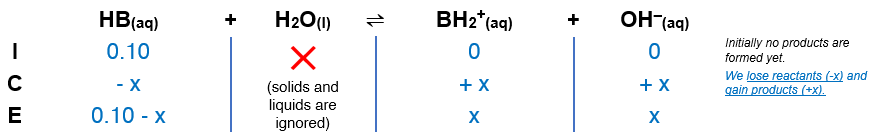# Problem: The pH of a 0.10 M solution of a weak base is 9.82. What is the Kb for this base?

###### FREE Expert Solution

We are being asked to calculate the Kb for a 0.1 M weak base (HB).

Step 1. Equilibrium reaction:        HB(aq) + H2O(l)   BH2+(aq) + OH-(aq)$\overline{){{\mathbf{K}}}_{{\mathbf{b}}}{\mathbf{=}}\frac{\mathbf{products}}{\mathbf{reactants}}{\mathbf{=}}\frac{\left[{\mathrm{BH}}_{2}\right]\left[{\mathrm{OH}}^{-}\right]}{\left[\mathrm{HB}\right]}}$

${\mathbf{K}}_{\mathbf{b}}\mathbf{=}\frac{\left[\mathbf{x}\right]\left[\mathbf{x}\right]}{\left[\mathbf{0}\mathbf{.}\mathbf{1}\mathbf{-}\mathbf{x}\right]}\phantom{\rule{0ex}{0ex}}\overline{){{\mathbf{K}}}_{{\mathbf{b}}}{\mathbf{=}}\frac{{\mathbf{x}}^{\mathbf{2}}}{\mathbf{0}\mathbf{.}\mathbf{1}\mathbf{-}\mathbf{x}}}$

100% (340 ratings)###### Problem Details

The pH of a 0.10 M solution of a weak base is 9.82. What is the Kb for this base?